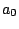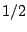Next: Controlling Amplitude Up: Sinusoids, amplitude and frequency Previous: Measures of Amplitude   Contents   Index

Units of Amplitude

Two amplitudes are often better compared using their ratio than their difference. Saying that one signal's amplitude is greater than another's by a factor of two might be more informative than saying it is greater by 30 millivolts. This is true for any measure of amplitude (RMS or peak, for instance). To facilitate comparisons, we often express amplitudes in logarithmic units called decibels. Ifis the amplitude of a signal (either peak or RMS), then we can define the decibel (dB) levelas:whereis a reference amplitude. This definition is set up so that, if we increase the signal power by a factor of ten (so that the amplitude increases by a factor of), the logarithm will increase by, and so the value in decibels goes up (additively) by ten. An increase in amplitude by a factor of two corresponds to an increase of about 6.02 decibels; doubling power is an increase of 3.01 dB. The relationship between linear amplitude and amplitude in decibels is graphed in Figure 1.3.Still usingto denote the reference amplitude, a signal with linear amplitude smaller thanwill have a negative amplitude in decibels:gives -20 dB,gives -40, and so on. A linear amplitude of zero is smaller than that of any value in dB, so we give it a dB level of.

In digital audio a convenient choice of reference, assuming the hardware has a maximum amplitude of one, isso that the maximum amplitude possible is 100 dB, and 0 dB is likely to be inaudibly quiet at any reasonable listening level. Conveniently enough, the dynamic range of human hearing--the ratio between a damagingly loud sound and an inaudibly quiet one--is about 100 dB.

Amplitude is related in an inexact way to the perceived loudness of a sound. In general, two signals with the same peak or RMS amplitude won't necessarily have the same loudness at all. But amplifying a signal by 3 dB, say, will fairly reliably make it sound about one step" louder. Much has been made of the supposedly logarithmic nature of human hearing (and other senses), which may partially explain why decibels are such a useful scale of amplitude [RMW02, p. 99].

Amplitude is also related in an inexact way to musical dynamic. Dynamic is better thought of as a measure of effort than of loudness or power. It ranges over nine values: rest, ppp, pp, p, mp, mf, f, ff, fff. These correlate in an even looser way with the amplitude of a signal than does loudness [RMW02, pp. 110-111].Next: Controlling Amplitude Up: Sinusoids, amplitude and frequency Previous: Measures of Amplitude   Contents   Index
Miller Puckette 2006-12-30